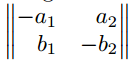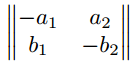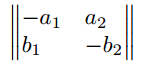# LaTeX Matrix

StartLaTeX Topicsfont sizearrowsminipageitalicsboldtext colormatrixfontslistingstables

## LaTeX Matrix

Within LaTeX there are several ways to set matrices and vectors. In the following commands and possibilities for the representation of matrices and vectors in LaTeX are shown.

### Standard LaTeX

However, within the standard there is no separate command or environment for this. Here you have to use the array environment if you want to set a matrix or a vector. But two things have to be considered, firstly the number and the alignment of the columns have to be defined and set in advance. On the other hand, the array environment lacks the typical parenthesis that encloses a matrix. This must be set additionally around the matrix. As an example for the representation of matrices the unit matrix is used here, since its structure might be generally known.

Input:
$\begin{array}{rrrr} 1 & 0 & 0 & 0 \\ 0 & 1 & 0 & 0 \\ 0 & 0 & 1 & 0 \\ 0 & 0 & 0 & 1 \\ \end{array}$

Output:
 \begin{array}{rrrr} 1 & 0 & 0 & 0 \\ 0 & 1 & 0 & 0 \\ 0 & 0 & 1 & 0 \\ 0 & 0 & 0 & 1 \\ \end{array}

In this simple example you can already see the problem with parentheses and that the columns have to be defined in advance. The parentheses can be inserted with the commands left and right, as the following examples show. The symbols in brackets must be a printable character or, if it is a special character like the braces {, }, the character must be masked.

Input:

$\left( \begin{array}{rrrr} 1 & 0 & 0 & 0 \\ 0 & 1 & 0 & 0 \\ 0 & 0 & 1 & 0 \\ 0 & 0 & 0 & 1 \\ \end{array}\right)$

$\left[ \begin{array}{rrrr} 1 & 0 & 0 & 0 \\ 0 & 1 & 0 & 0 \\ 0 & 0 & 1 & 0 \\ 0 & 0 & 0 & 1 \\ \end{array}\right]$

Output:
 $\left( \begin{array}{rrrr} 1 & 0 & 0 & 0 \\ 0 & 1 & 0 & 0 \\ 0 & 0 & 1 & 0 \\ 0 & 0 & 0 & 1 \\ \end{array}\right)$ $\left[ \begin{array}{rrrr} 1 & 0 & 0 & 0 \\ 0 & 1 & 0 & 0 \\ 0 & 0 & 1 & 0 \\ 0 & 0 & 0 & 1 \\ \end{array}\right]$

Using the commands cdots, ddots and vdots, omissions, i.e. dots, within the matrix between columns and rows are also possible.

Input:
$\left( \begin{array}{rrrr} 1 & 0 & \cdots & 0 \\ 0 & \ddots & 0 & \vdots \\ \vdots & 0 & \ddots & 0 \\ 0 & \cdots & 0 & 1 \\ \end{array}\right)$

Output:
 $\left( \begin{array}{rrrr} 1 & 0 & \cdots & 0 \\ 0 & \ddots & 0 & \vdots \\ \vdots & 0 & \ddots & 0 \\ 0 & \cdots & 0 & 1 \\ \end{array}\right)$

### amsmath usepackage

If the amsmath usepackage is included, there are six different matrix environments available. The only difference between the environments is the respective parentheses of the matrices.

Another difference to the array environment is that the number of columns no longer has to be specified. The number of columns used is automatically recognized. In case a matrix with more than 10 columns is used, you will get the following error message.

! Extra alignment tab has been changed to \cr.


A possible solution is to set the counter to a higher value by \setcounter{MaxMatrixCols}{new value} this command changes the value of the maximum number of columns.

#### matrix without brackets

 input output $\begin{matrix} a_1 & a_2 & a_3 \\ b_1 & b_2 & b_3 \\ c_1 & c_2 & c_3 \end{matrix}$#### matrix with parentheses brackets

 input output $\begin{pmatrix} a_1 & a_2 & a_3 \\ b_1 & b_2 & b_3 \\ c_1 & c_2 & c_3 \end{pmatrix}$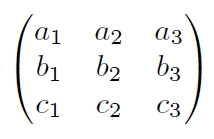#### matrix with brackets

 input output $\begin{bmatrix} a_1 & a_2 & a_3 \\ b_1 & b_2 & b_3 \\ c_1 & c_2 & c_3 \end{bmatrix}$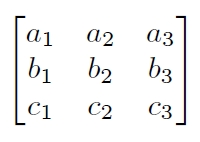#### matrix with curly brackets

 input output $\begin{Bmatrix} a_1 & a_2 & a_3 \\ b_1 & b_2 & b_3 \\ c_1 & c_2 & c_3 \end{Bmatrix}$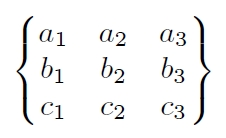#### matrix with vertical bar brackets

 input output $\begin{vmatrix} a_1 & a_2 & a_3 \\ b_1 & b_2 & b_3 \\ c_1 & c_2 & c_3 \end{vmatrix}$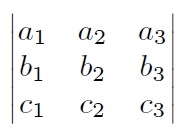#### matrix with double vertical bar brackets

 input output $\begin{Vmatrix} a_1 & a_2 & a_3 \\ b_1 & b_2 & b_3 \\ c_1 & c_2 & c_3 \end{Vmatrix}$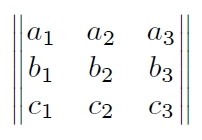Another difference between the environments of amsmath and the array environment is that the orientation of the columns cannot be changed, which is not noticeable in the representation of the unit matrix. One package that allows the columns to be aligned is the mathtools usepackage. This package will be discussed later.

Besides the environments for normal matrices, the amsmath usepackage also provides an environment for setting matrices within continuous text -- the smallmatrix environment. This environment does not bring brackets per se, but as with the array environment, the brackets can also be manually inserted here.

This text  $\begin{smallmatrix} a&b\\ c&d \end{smallmatrix}$ is only for showing.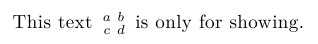This text  $\bigl( \begin{smallmatrix} a&b\\ c&d \end{smallmatrix} \bigr)$ is only for showing.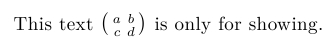For the omissions there is a new command hdotsfor with which at least horizontal dots can be set.

$\begin{pmatrix} 1 & 0 & \cdots & \cdots & 0 \\ 0 & \ddots & \vdots & 0 & \vdots \\ \vdots & \vdots & \ddots & 0 & \vdots \\ \vdots & \vdots & 0 & \ddots & \vdots \\ 0 & \cdots & \cdots & 0 & 1 \\ \end{pmatrix}$

$\begin{pmatrix} 1 & 0 & \hdotsfor{2} & 0 \\ 0 & \ddots & \vdots & 0 & \vdots \\ \vdots & \vdots & \ddots & 0 & \vdots \\ \vdots & \vdots & 0 & \ddots & \vdots \\ 0 & \hdotsfor{2} & 0 & 1 \\ \end{pmatrix}$

Output:
 \begin{pmatrix} 1 & 0 & \cdots & \cdots & 0 \\ 0 & \ddots & \vdots & 0 & \vdots \\ \vdots & \vdots & \ddots & 0 & \vdots \\ \vdots & \vdots & 0 & \ddots & \vdots \\ 0 & \cdots & \cdots & 0 & 1 \\ \end{pmatrix}### mathtools

The mathtools usepackage extends the amsmath usepackage's matrix environments with the ability to set column alignment. It is now possible to use right-aligned or left-aligned columns within the matrix environments.

The structure corresponds to the environments from amsmath, but the names of the environments have an additional star at the end. The desired orientation is passed as options r (right justified), c (centered) or l (left justified). However, it must be said that only all columns can be aligned uniformly. In the case that a matrix is desired in which different columns have a different alignment, this can be realized via the array environment.

The mathtools usepackage also offers adaptations for the matrices in the continuous text.

#### Matrix without brackets

 $\begin{matrix*}[r] -a_{1} & a_{2} \\ b_{1} & -b_{2} \end{matrix*}$  $\begin{matrix*}[c] -a_{1} & a_{2} \\ b_{1} & -b_{2} \end{matrix*}$  $\begin{matrix*}[l] -a_{1} & a_{2} \\ b_{1} & -b_{2} \end{matrix*}$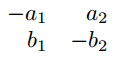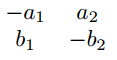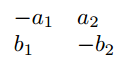#### Matrix with parentheses

 $\begin{pmatrix*}[r] -a_{1} & a_{2} \\ b_{1} & -b_{2} \end{pmatrix*}$  $\begin{pmatrix*}[c] -a_{1} & a_{2} \\ b_{1} & -b_{2} \end{pmatrix*}$  $\begin{pmatrix*}[l] -a_{1} & a_{2} \\ b_{1} & -b_{2} \end{pmatrix*}$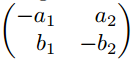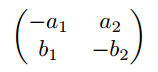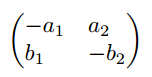#### Matrix with brackets

 $\begin{bmatrix*}[r] -a_{1} & a_{2} \\ b_{1} & -b_{2} \end{bmatrix*}$  $\begin{bmatrix*}[c] -a_{1} & a_{2} \\ b_{1} & -b_{2} \end{bmatrix*}$  $\begin{bmatrix*}[l] -a_{1} & a_{2} \\ b_{1} & -b_{2} \end{bmatrix*}$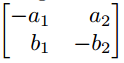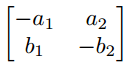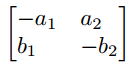#### Matrix with curly brackets

 $\begin{Bmatrix*}[r] -a_{1} & a_{2} \\ b_{1} & -b_{2} \end{Bmatrix*}$  $\begin{Bmatrix*}[c] -a_{1} & a_{2} \\ b_{1} & -b_{2} \end{Bmatrix*}$  $\begin{Bmatrix*}[l] -a_{1} & a_{2} \\ b_{1} & -b_{2} \end{Bmatrix*}$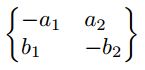#### Matrix with vertical line (absolute line or determinant matrix).

 $\begin{vmatrix*}[r] -a_{1} & a_{2} \\ b_{1} & -b_{2} \end{vmatrix*}$  $\begin{vmatrix*}[c] -a_{1} & a_{2} \\ b_{1} & -b_{2} \end{vmatrix*}$  $\begin{vmatrix*}[l] -a_{1} & a_{2} \\ b_{1} & -b_{2} \end{vmatrix*}$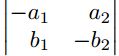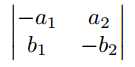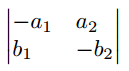#### Matrix with double vertical line (norm matrix).

 $\begin{Vmatrix*}[r] -a_{1} & a_{2} \\ b_{1} & -b_{2} \end{Vmatrix*}$  $\begin{Vmatrix*}[c] -a_{1} & a_{2} \\ b_{1} & -b_{2} \end{Vmatrix*}$  $\begin{Vmatrix*}[l] -a_{1} & a_{2} \\ b_{1} & -b_{2} \end{Vmatrix*}$